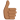# Roller-coaster problem

• Lis

#### Lis

Moved from technical forums so no template
1. In an amusement park, the new roller-coaster ride The Loopy Looper is com- posed of a number of full loops, where the car goes round upside down. The car is attached to the track, but there are no seat belts. Consider one such loop, which can be taken as a circle with radius r. The mass of the roller-coaster car is M, including the weight of the people in it, and you may ignore all sources of friction. The car starts out at ground level with initial speed vi, and after that the engine is turned off. There is gravity g.

a) What is the minimum value of vi, for the car to get to the top of the loop, assuming that the passengers hold on to something, to not fall out?

F
s=G

m(v^2/r)=mg

v^2/r=g

v=sqrt(g*r)

Am I thinking right?

The velocity you have calculated is the minimum required speed 'at the top of the loop'. Are the speeds at the ground level and the top level equal? [Hints: Use energy conservation.]

1/2mvi^2 = 1/2mvf^2

vi^2=sqrt(r*g)

Is that correct?

1/2mvi^2 = 1/2mvf^2

vi^2=sqrt(r*g)

Is that correct?
You have forgot to consider the potential energy term.

ops, ok i´ll try again:

1/2mvi^2 + mghi = 1/2mv^2 + mghf

Multiplyng by 2

mvi^2= mv^2 + 2(mghf)

vi=sqrt(mv^2 + 2(mghf))

ops, ok i´ll try again:

1/2mvi^2 + mghi = 1/2mv^2 + mghf

Multiplyng by 2

mvi^2= mv^2 + 2(mghf)

vi=sqrt(mv^2 + 2(mghf))

Exactly!•Lis
Great!
I could also need some help in b:

b) What is the minimum value of vi, for the car to get to the top of the loop, and for the people to not fall out as it goes round, even when not holding on to anything?

Can you give me some hints here as well?

Oh, sorry! I missed the line.
assuming that the passengers hold on to something
If the passenger hold on to something, he does not need to have any velocity at the top of the loop to not fall out. At the ground level, he just needs to have kinetic energy = mass * g * height of the top of the loop
The problem we have solved earlier is for the case when the passenger does not hold on to something.
Sorry once again for my mistake.

Okey so the answer i solved belongs to the problem where the passenger does not hold on to something?

So fore the case where the passengers is holding on:

vi= sqrt(2gh)

Correct?

Okey so the answer i solved belongs to the problem where the passenger does not hold on to something?

So fore the case where the passengers is holding on:

vi= sqrt(2gh)

Correct?
Exactly!

Great! Thank you :-)

vi=sqrt(mv^2 + 2(mghf))
That's dimensionally wrong, with masses on one side but not the other.
The original question specified r, no need to change that to hf.
You need to combine this with your earlier equation to get rid of the reference to v (vf) on the right.

I totally agree with you. Thank you! I was running out of time earlier so that might be the reason why i did those silly mistakes.